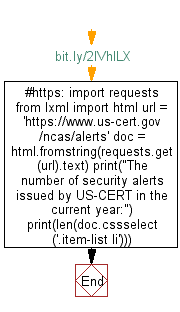# Python Web Scraping: Get the number of security alerts issued by US-CERT in the current year

## Python Web Scraping: Exercise-14 with Solution

Write a Python program to get the number of security alerts issued by US-CERT in the current year.

Sample Solution:-

Python Code:

``````#https://bit.ly/2lVhlLX
import requests
from lxml import html
doc = html.fromstring(requests.get(url).text)
print("The number of security alerts issued by US-CERT in the current year:")
print(len(doc.cssselect('.item-list li')))
```
```

Output

```The number of security alerts issued by US-CERT in the current year:
37
```

Flowchart:Python Code Editor:

Have another way to solve this solution? Contribute your code (and comments) through Disqus.

What is the difficulty level of this exercise?

﻿

## Python: Tips of the Day

Python: The Zip() Function

```>>> students = ('John', 'Mary', 'Mike')
>>> ages = (15, 17, 16)
>>> scores = (90, 88, 82, 17, 14)
>>> for student, age, score in zip(students, ages, scores):
...     print(f'{student}, age: {age}, score: {score}')
...
John, age: 15, score: 90
Mary, age: 17, score: 88
Mike, age: 16, score: 82
>>> zipped = zip(students, ages, scores)
>>> a, b, c = zip(*zipped)
>>> print(b)
(15, 17, 16)
```